# Range: validate then process

Tags

### The pattern

Here's what we did in the previous lesson.

• For each record:
•   Grab the record's data from the Range
•   Normalize the data
•   Validate the data
•   Process the data

Let's add to the last step. We'll keep it simple: computing the average of valid data.

### Goat cuteness data

Cthulhu has some data on goat cuteness it wants you to analyze. Here's part of the start worksheet: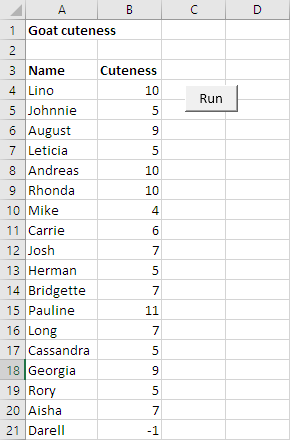Cuteness should be a number, from 1 to 10.

When the Run button is pressed, show average cuteness, for valid data only.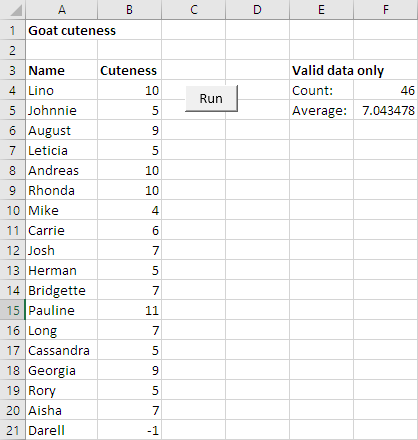### Code outline

Let's start by planning the code. Here's what we'll do:

• For each record:
•   Grab the record's data from the Range
•   Normalize the data
•   Validate the data
•   Process the data

There isn't any normalization to do. Also, we'll just validate the cuteness field, not name.

Here's some comments to help us on the way:

• Option Explicit
•
• Sub run()
•
•     'Initialize.
•
•     'Create the Range.
•
•     'Loop over records.
•
•         'Get fields for current record
•
•         'Initialize validation flag.
•
•         'Validate
•
•         'Is the data valid?
•
•     'Compute average.
•
•     'Output.
•
• End Sub

This is what we did in the last lesson.

### Initialize

To compute an average, we need a total, and a count. Let's make those variables, and initialize them. Also, let's create the Range.

• Sub run()
•     Dim rData As Range
•     Dim sNumberOfRecords As Single
•     Dim sNumberOfValidRecords As Single
•     Dim sTotalCutenessValidOnly As Single
•     Dim sAverageCutenessValidOnly As Single
•
•     'Initialize.
•     sNumberOfValidRecords = 0
•     sTotalCutenessValidOnly = 0
•
•     'Create the Range.
•     Set rData = Range(Cells(4, 1), Cells(4, 1).End(xlToRight).End(xlDown))
•     sNumberOfRecords = rData.Rows.Count

Let's name the variables to remind ourselves that we're doing. Instead of `sTotalCuteness`, use `sTotalCutenessValidOnly`. This is optional, but if it helps you, do it.

OK, add the loop, and the code that reads in the current record. I'm going to start leaving out code you've already seen. You can download the solution to see it all.

• Sub run()
•     ...
•     Dim sRecordNumber As Single
•     Dim tUncheckedCurrentCuteness As String
•     Dim tCurrentName As String
•     Dim sCurrentCuteness As Single
•     ...
•
•     Const FIELD_NAME = 1
•     Const FIELD_CUTENESS = 2
•
•     'Initialize.
•     sNumberOfValidRecords = 0
•     sTotalCutenessValidOnly = 0
•
•     'Create the Range.
•     Set rData = Range(Cells(4, 1), Cells(4, 1).End(xlToRight).End(xlDown))
•     sNumberOfRecords = rData.Rows.Count
•
•     'Loop over records.
•     For sRecordNumber = 1 To sNumberOfRecords
•         'Get fields for current record
•         tCurrentName = rData.Cells(sRecordNumber, FIELD_NAME)
•         tUncheckedCurrentCuteness = rData.Cells(sRecordNumber, FIELD_CUTENESS)
•
•     Next sRecordNumber

Notice the `Consts`. They make the later code easier to read, and maintain.

### Validation

We'll only validate the cuteness field. Let's use the same approach as before, with the flag pattern.

• 'Initialize validation flag.
• tIsDataOk = "yes"
• 'Validate
• If Not IsNumeric(tUncheckedCurrentCuteness) Then
•     tIsDataOk = "no"
• Else
•     sCurrentCuteness = tUncheckedCurrentCuteness
•     If sCurrentCuteness < 1 Or sCurrentCuteness > 10 Then
•         tIsDataOk = "no"
•     End If
• End If
• 'Is the data valid?
• If tIsDataOk = "yes" Then
•     'Processing.
•
• End If

### Processing

We want to have a count and total. If the data is valid, add to total and count variables.

1. Option Explicit
2.
3. Sub run()
4.     ...
5.     'Initialize.
6.     sNumberOfValidRecords = 0
7.     sTotalCutenessValidOnly = 0
8.     ...
9.     'Loop over records.
10.     For sRecordNumber = 1 To sNumberOfRecords
11.         'Get fields for current record
12.         ...
13.         tUncheckedCurrentCuteness = rData.Cells(sRecordNumber, FIELD_CUTENESS)
14.         '(Move into sCurrentCuteness if valid)
15.         ...
16.         'Is the data valid?
17.         If tIsDataOk = "yes" Then
18.             'Processing.
19.             sNumberOfValidRecords = sNumberOfValidRecords + 1
20.             sTotalCutenessValidOnly = sTotalCutenessValidOnly + sCurrentCuteness
21.         End If
22.     Next sRecordNumber
23.     ...
24. End Sub

Lines 6 and 7 initialize variables for count and total.

Lines 10 to 22 loop over records. Line 13 gets a cuteness value for the current record. If it's valid, line 19 increases the count of valid records by one. Line 20 increases the total, by the new cuteness value.

### Average and output

OK, after the loop ends, we'll have the count and total we need to compute the average.

• 'Compute average.
• sAverageCutenessValidOnly = sTotalCutenessValidOnly / sNumberOfValidRecords
•
• 'Output.
• Cells(4, 6) = sNumberOfValidRecords
• Cells(5, 6) = sAverageCutenessValidOnly

That's it! Remember, you can download the solution, and trace it with the debugger.Adela

A question. What if all of the data is invalid? Count will still be zero after the loop ends, right?

Oh, wow! Great thinking! Here's what Adela means:

Option Explicit

• Sub run()
•     ...
•     'Initialize.
•     sNumberOfValidRecords = 0
•     sTotalCutenessValidOnly = 0
•     ...
•     'Loop over records.
•     For sRecordNumber = 1 To sNumberOfRecords
•         ...
•         'Is the data valid?
•         If tIsDataOk = "yes" Then
•             'Processing.
•             sNumberOfValidRecords = sNumberOfValidRecords + 1 Never happens!
•             sTotalCutenessValidOnly = sTotalCutenessValidOnly + sCurrentCuteness
•         End If
•     Next sRecordNumber
•     sNumberOfValidRecords is still 0
•     'Compute average.
•     sAverageCutenessValidOnly = sTotalCutenessValidOnly / sNumberOfValidRecords
•     Crashy crashy
•     ...
• End Sub

`sNumberOfValidRecords` starts at 0. If all of the data is invalid it never increases. It's still 0 at the end of the loop.

What happens when you divide by 0? Crashy crashy.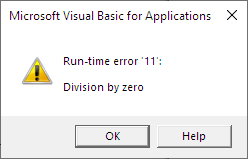You could use an `If` to allow for this:

• Next sRecordNumber
• 'Guard against no valid data.
• If sNumberOfValidRecords = 0 Then
•     Cells(4, 6) = "No valid records. Cannot compute average."
•     End
• End If
• 'Compute average.
• sAverageCutenessValidOnly = sTotalCutenessValidOnly / sNumberOfValidRecords
•
• 'Output.
• Cells(4, 6) = sNumberOfValidRecords
• Cells(5, 6) = sAverageCutenessValidOnly

This is the guard pattern at work.

Pattern

Prevent your code from doing something crazy.

### Exercises

Exercise

Goat weight program

Cthulhu thinks its goat friends are looking a little thin, so it sponsors a weight gain program. Goats are weighed at the start and end of a week. The more weight gained, the better!

You can download the start workbook. It looks like this: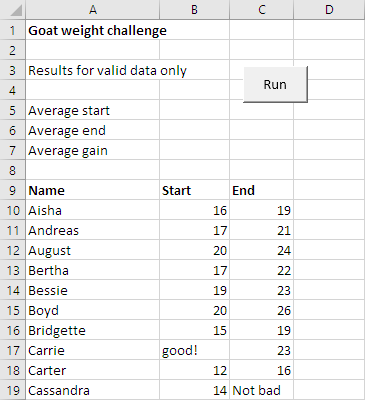Write a program that computes the average start and end weights, and the average gain. Only include valid data. The names are all OK, so you don't need to check them. However, as you can see, there's some bad numeric data. Exclude it from the analysis. Also exclude negative weights. No floating goats!

Here's what the output should look like: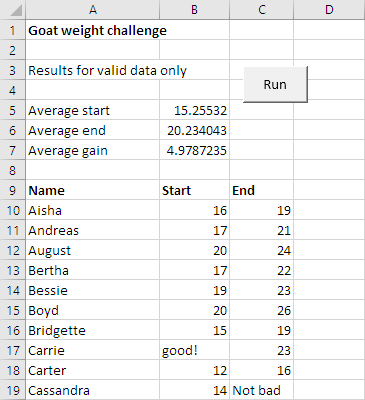Upload your solution. The usual standards apply.

Exercise

Goat ball spread

Cthulhu is thinking about betting on goat ball point spreads. It wants you to figure out come statistics.

You can download the start workbook with the data. It looks like this: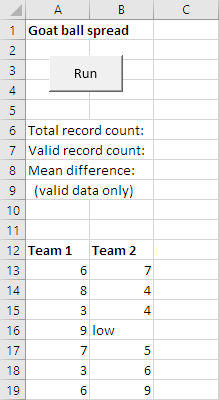The scores should all be numbers that are zero or more. There are data errors.

Write a program to compute the two counts and the mean, as well as the score differences. Here's the output: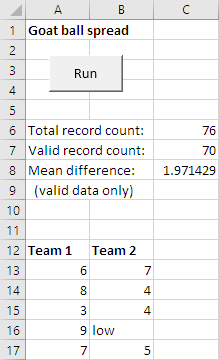Differences are always positive, or zero.

You can assume that at least some of the data is valid.

Upload your solution. The usual standards apply.

Exercise

Bouncy boots

Goats love to bounce. Like Tiggers.Cthulhu got its goaty friends bouncy boots. Do the boots work? Write a program to find out.

Your worksheet should start like this: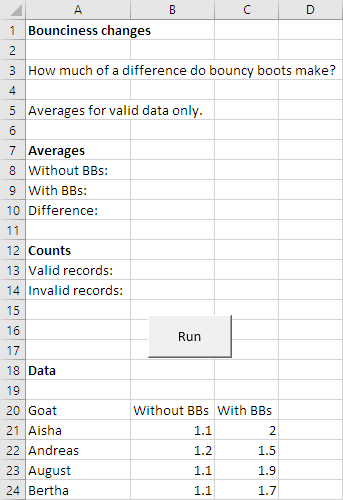You can download the start worksheet.

Each data row has three values:

• Goat name. Should not be blank. A name that is just spaces counts as blank.
• Height goat jumps, without bouncy boots (meters). Should be a number that's zero or more.
• Height goat jumps, with bouncy boots (meters). Should be a number that's zero or more.

Your program should validate the data, and show some statistics.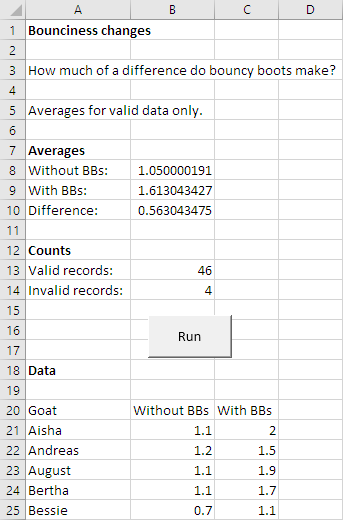Upload your solution. The usual standards apply.

Exercise

Database lesson

Goat love databases. They like nothing better than writing SQL queries.

Cthulhu teaches an SQL class. It had an idea for improving a lesson. It gave some goats the old lesson, then a test (the "before" test), then the new lesson, then another test (the "after" test). What happened to the scores?

Download the starting worksheet with the data. It's like this: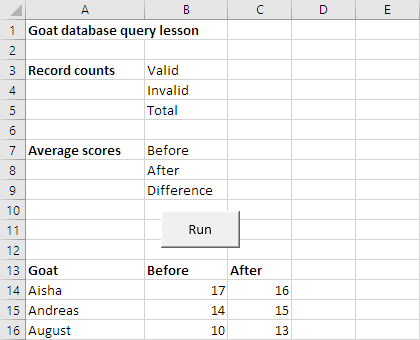Notice that scores can go down. That's normal; scores have some randomness to them.

Each record has three values:

• Name. Cannot be empty.
• Before. Number, zero or more.
• After. Number, zero or more.

Some data might not fit the rules. You'll need to validate it.

Write a program to compute averages and counts. Here's the output.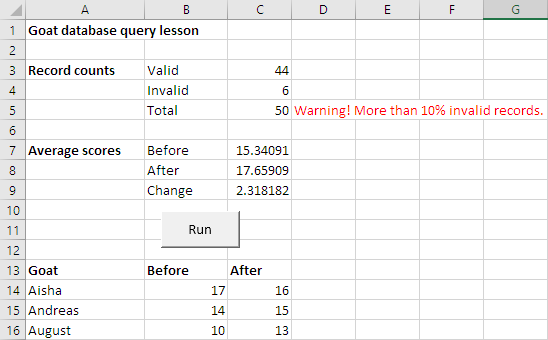Your program should warn the user if more than 10% of the records are invalid. Don't forget to remove the message each time the program runs.

Upload your solution. The usual standards apply.

Exercise

Supernatural scoresSupernatural is Cthulhu's fave workout app, for the Oculus Quest. That's Coach Doc, lunging to hit some virtual targets.

Some of Cthulhu's goaty friends use the app, too. Each workout is scored on accuracy and power, numbers from 0 to 100. The workout's combined score is the average of the two.

Write a program to help Cthulhu see how the goats are doing.

Here's how the worksheet looks at the beginning. You can download it.You can see the data at the bottom. The number of records could change each time the program runs, so allow for that in your code. Assume there are no errors in the data.

The user types a minimum number in cell B3. Your program will include only records with a combined score above that value. For example, if the user typed 84, the program would include records with a combined score above 84.

Remember, the combined score is the average of accuracy and power. So if accuracy is 90 and power is 86, the combined score will be 88.

Validate the minimum value entered by the user. It must be numeric, more than 1, and less than 100. If there's an error, show a message to the right of the input, in red (and stop the program):Be sure to erase the error message cell, and the output, each time the program runs.

Here's some output:So 24 records had combined scores above 85. The average combined score for those records was 90.22916.

Another example:In this case, there were no records with a combined score above 99. Trying to compute an average when the count is zero will give an overflow error. So, if the count is 0, show "No data" as the mean.

Remember:

• The number of records could change.
• Clear output at the start of each run.
• Test combined scores against the minimum.
• Assume there are no errors in the data.
• Don't try to calculate the mean if no records pass the minimum combined score test.

Upload your solution. The usual coding standards apply.

### Up next

OK, that's it for `Range`s for a while. Let's move on to reading data from CSV files.

Attachments